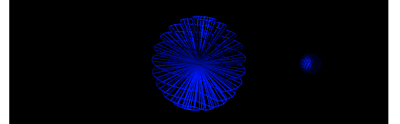## Monday, June 11, 2012

‹prev | My Chain | next›

Having discovered (that is, been told) that Gladius does not yet support CubicVR.js primitives, I set out last night to generate a sphere procedurally. I got as far as making a circle:To build that, I work around 24 slices of 2π radians / 360°, calculating the X-Y position with sines and cosines. I then build a face from two slices and the origin (0, 0, 0):
``````  for (var j=1; j<=24; j++) {
points[j] = [
Math.cos(M_TWO_PI * j/24),
Math.sin(M_TWO_PI * j/24),
0
];
if (j > 1) {
faces[j-1] = [0, j, j-1];
}
}``````
To make a sphere, I need to do something with the Z coordinate instead of always setting it to zero. As I move from pole (0, 0, -1) to pole (0, 0, 1), I also need to make sure that the X and Y coordinates get close to the origin. This can again be accomplished with simple sines and cosines along with the introduction of another loop:
``````  var index = 0;
for (var i=0; i<12; i++) {
for (var j=1; j<24; j++) {
index = index +1;
points[index] = [
Math.sin(Math.PI * i/12) * Math.cos(M_TWO_PI * j/24),
Math.sin(Math.PI * i/12) * Math.sin(M_TWO_PI * j/24),
Math.cos(Math.PI * i/12)
];
if (index > 0) {
faces[index-1] = [0, index, index-1];
}
}
}``````
With that, I have a sphere. Well, almost:The mesh is still comprised of "faces" that start at the center of the sphere and include two points on the outside of the sphere. If I disable the wireframing, then I see that I have no outside faces. Rather I have a spiral inside the sphere:Building the faces actually turns out to be a bit of pain. I had expected to build square faces all the way around. But something in the UV mapper only saw faces with three corners. I eventually give in and, for each square, I assemble two faces:
``````  for (var i=0; i<12; i++) {
for (var j=0; j<24; j++) {
points.push([
Math.sin(Math.PI * i/12) * Math.cos(M_TWO_PI * j/24),
Math.sin(Math.PI * i/12) * Math.sin(M_TWO_PI * j/24),
Math.cos(Math.PI * i/12)
]);
if (points.length > 25) {
var idx = points.length-1;
faces.push([
idx-25,
idx-24,
idx
]);
faces.push([
idx-25,
idx,
idx-1
]);
}
}
}``````
With that, I get my sphere:That was a pain. Especially needing to build that up by triangles. I may need to spend some time another day to figure out why that was needed.

Day #414